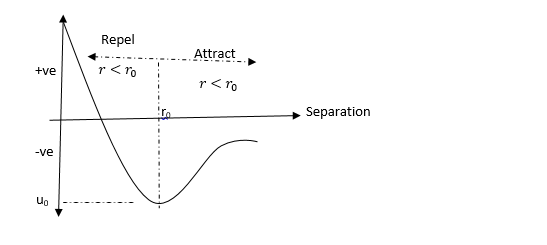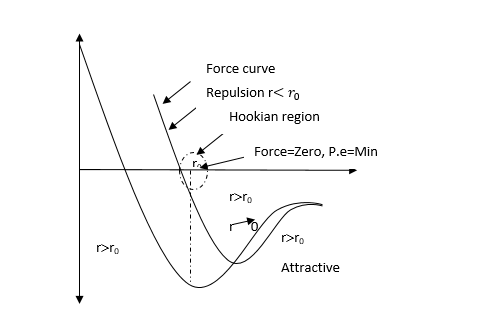# Relationship between Intermolecular potential energy and Particle Separations.

In this page we will are going to explore the Relationship between Intermolecular Potentail Engergy [P.E] and Particle Separations. we are also going to see how the P.E Curve to explain some properties of Material, This is a continuation of our Matter series , topic sen in the gcse and gce A level.

## Intermolecular potential energy [P.E]

2 molecules would repel and attract each other depending of their separation. When far apart their P.E due to interaction is 0. When close to each other, their P.E due to interaction action between them until they reach the equilibrium separation r0 where the overall force is 0 if the molecules are pushed closer the P.E increases as work is done on the repulsive forces due to the electron.Intermolecular potential energy curveIntermolecular potential energy and Particle Separations

### Using the Intermolecular potential energy curve to explain some properties of Material

I – Surface Tension

Molecules inside the liquid are at equilibrium separation ro i.e. all forces on them are O. Moles near or at the surface are more spaced out i.e. Mro and the net force on them is attractive (even though weak) this moles are thus in a state of tension thus a need i.e. can be made to lie on the surface of water.

ii Hooke’s law

The intermolecular force separation curve is straight at and near the equilibrium separation ro the straight nature and near ro means the separation is proportionate to the force which is hooke’s law provided the separation is not too much to cause permanent stretching. Thus hooker’s law is obeyed only within this region “Hookian region”

iii) Expansion

At normal temperature the intermolecular separation is minimum which corresponds to o and ro suppose the subs is given some energy ΔU. The energy of the system = μo + ΔU  Moves from  μo  to  μ. This causes the separation of the particles to have a shift to the right of their equilibrium position. The new equilibrium position is greater the r0 r>r0 . Because of the asymmetric nature of the graph. This means the amplitude of variation of the particles increases. The net effect for all the particles increases. The net effect for all the particles that makeup the material is an increase in size of the material. Hence materials expand when heated.

iv) Latent heat

it can be seen from p.e separation curve that if 2 atoms are to become completely free from each other’s influence to acquire O potential energy of such. Then they need to gain an amount  of energy. Thus ξ0   can be considered to be the binding energy of such a pair of atom consider a solid in which each atom has n nearest neighbors that is a coordination number of n). Since interatomic forces at short range, it can be assumed that each of the 2 atoms involve in  n bond. In other for such a bond to be broken, the amount of energy that each of the atoms interact only with these n atoms that each of the 2 atoms has to acquire is  ξ/2,  since the change of atoms in 1 mole is numerically equal Na and each atom is involved in n bonds, the total energy require to separate the atoms of 1 mole of solid completely at absolute